# Common Core: High School - Geometry : Rotation, Reflection, and Transformation Definitions: CCSS.Math.Content.HSG-CO.A.4

## Example Questions

← Previous 1

### Example Question #1 : Rotation, Reflection, And Transformation Definitions: Ccss.Math.Content.Hsg Co.A.4

Two pencils are placed equidistance away from one another on a table. If these two pencils are parallel, what is the result after they are reflected.

The two pencils are now perpendicular.

The two pencils do not move.

The two pencils are located on the same line.

The two pencils are still parallel.

The two pencils are still parallel.

Explanation:

Before solving this problem recall the definition of a reflection.

Reflection: The transformation of each and every point on a line or figure across some line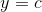whereis some constant or linear function.

Applying this definition to the situation at hand result in the following depiction. The pencils are represented by the blue and black lines and the reflection line is the red dashed line. After the reflection occurs, the pencils essentially swap positions. Since the pencils were parallel to start with, they remain parallel after the reflection occurred.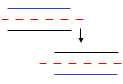"The two pencils are still parallel."

### Example Question #2 : Rotation, Reflection, And Transformation Definitions: Ccss.Math.Content.Hsg Co.A.4

Given a circle that is divided intoequal pieces, what is the number of rotations that can occur to keep symmetry?

Three

Six

Twelve

Five

Six

Explanation:

Drawing the described circle is the first step in answering this given question.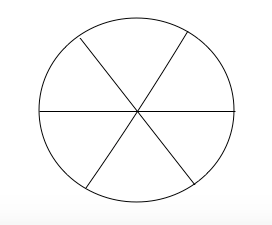To calculate the number of symmetrical rotations, take one piece and rotate it clockwise until it results in the exact image as originally started with. Since the circle is divided into six equal pieces that means that rotating one of the pieces can be done six different times and still keep rotational symmetry, which also means keeping the angles of each piece the same and returning to the original image.

Therefore the correct answer is six.

### Example Question #1 : Rotation, Reflection, And Transformation Definitions: Ccss.Math.Content.Hsg Co.A.4

Given a regular polygon that hassides, calculate the order of rotational symmetry.Explanation:

For this particular question recall what rotational symmetry means. For an object to achieve rotational symmetry it must return to its original form. This can be accomplished by rotating the figure or equal parts of the figure in either the clockwise or non clockwise direction.

For this particular problem, it is stated that the polygon is "regular" meaning all sides and angles are equal to one another. It is also said that the polygon hassides. This means that the figure can be rotatedtimes to return to its original form.

Therefore, to obtain rotational symmetry of a regular polygon, it must have an order equal to the number of sides.

Thus, the answer is.

### Example Question #1 : Rotation, Reflection, And Transformation Definitions: Ccss.Math.Content.Hsg Co.A.4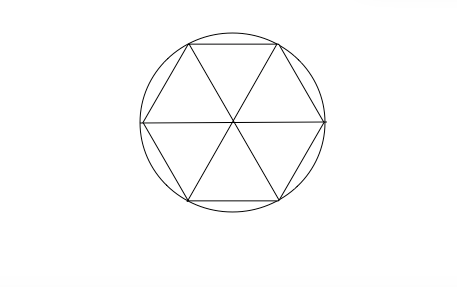Looking at the above image, calculate the order of rotational symmetry of the polygon that is inscribed in the circle.Explanation:

For this particular question recall what rotational symmetry means. For an object to achieve rotational symmetry it must return to its original form. This can be accomplished by rotating the figure or equal parts of the figure in either the clockwise or non clockwise direction.

For this particular problem, look at the following image.The polygon has six sides. This means that the figure can be rotatedtimes to return to its original form.

Therefore, to obtain rotational symmetry of a regular polygon, it must have an order equal to the number of sides.

Thus, the answer is.

### Example Question #2 : Rotation, Reflection, And Transformation Definitions: Ccss.Math.Content.Hsg Co.A.4

For an equilateral triangle that is inscribed in a circle, what is the minimum angle rotation that can be done to result in the same triangle orientation?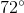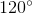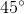Explanation:

Recall that the circle isdegrees. It is also important to recall that an equilateral triangle is a triangle whose side lengths are equal and the angles are equal. When the triangle is inscribed in the circle three lines can be drawn from the vertex on each point to the midpoint of the opposite side. This results in the following image.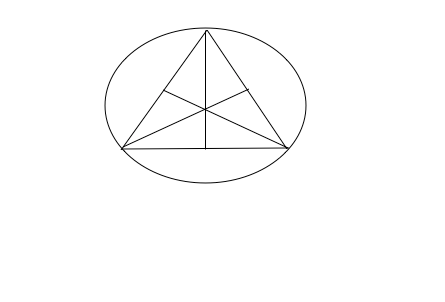From here, calculate the central angle between two points of the triangle. Since the circle isdegrees and the triangle is divided into six equal sectors then each sector isdegrees. Now, there are two sectors between two points of the triangle. Therefore, the minimum angle rotation that can be done to result in the same triangle isdegrees.

### Example Question #2 : Rotation, Reflection, And Transformation Definitions: Ccss.Math.Content.Hsg Co.A.4

Given a circle that is divided intoequal pieces, what is the number of rotations that can occur to keep symmetry?Explanation:

To calculate the number of symmetrical rotations, take one piece and rotate it clockwise until, it results in the exact image as originally started with. Since the circle is divided intoequal pieces that means that rotating one of the pieces can be done two different times and still keep rotational symmetry, which also means keeping the angles of each piece the same and returning to the original image.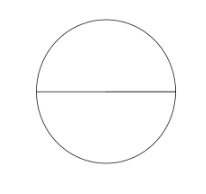Therefore the correct answer is.

### Example Question #41 : High School: Geometry

Given a circle that is divided intoequal pieces, what is the number of rotations that can occur to keep symmetry?Explanation:

To calculate the number of symmetrical rotations, take one piece and rotate it clockwise until, it results in the exact image as originally started with. Since the circle is divided intoequal pieces that means that rotating one of the pieces can be done 8 different times and still keep rotational symmetry, which also means keeping the angles of each piece the same and returning to the original image.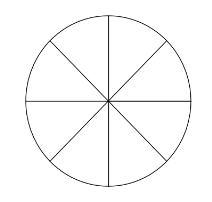Therefore the correct answer is.

### Example Question #41 : High School: Geometry

Given a circle that is divided intoequal pieces, what is the number of rotations that can occur to keep symmetry?Explanation:

To calculate the number of symmetrical rotations, take one piece and rotate it clockwise until, it results in the exact image as originally started with. Since the circle is divided into 16 equal pieces that means that rotating one of the pieces can be donedifferent times and still keep rotational symmetry, which also means keeping the angles of each piece the same and returning to the original image.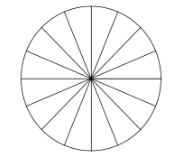Therefore the correct answer is.

### Example Question #1 : Rotation, Reflection, And Transformation Definitions: Ccss.Math.Content.Hsg Co.A.4

Given a circle that is divided intoequal pieces, what is the number of rotations that can occur to keep symmetry?Explanation:

To calculate the number of symmetrical rotations, take one piece and rotate it clockwise until, it results in the exact image as originally started with. Since the circle is divided intoequal pieces that means that rotating one of the pieces can be donedifferent times and still keep rotational symmetry, which also means keeping the angles of each piece the same and returning to the original image.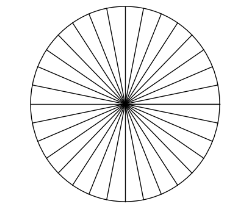Therefore the correct answer is.

### Example Question #8 : Rotation, Reflection, And Transformation Definitions: Ccss.Math.Content.Hsg Co.A.4

Given a circle that is divided intoequal pieces, what is the number of rotations that can occur to keep symmetry?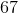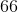Explanation:

To calculate the number of symmetrical rotations, take one piece and rotate it clockwise until, it results in the exact image as originally started with. Since the circle is divided intoequal pieces that means that rotating one of the pieces can be donedifferent times and still keep rotational symmetry, which also means keeping the angles of each piece the same and returning to the original image.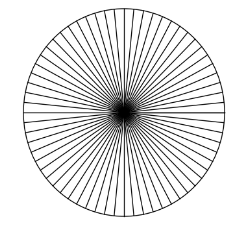Therefore the correct answer is.

← Previous 1

### All Common Core: High School - Geometry Resources